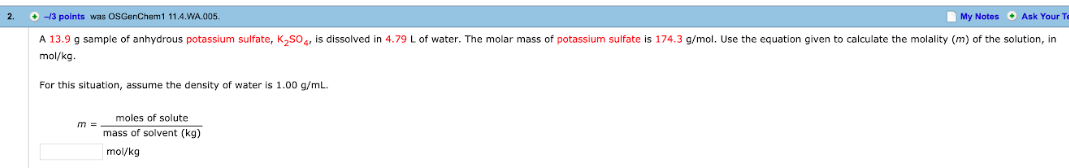# 2. A 13.9 g sample of anhydrous potassium sulfate K2SO4 is dissolved in 4.79 L of water. The molar mass of potassium sulfate is 174.3 g/mol. Use the equation given to calculate given to calculate the molality (m) of the solution, in mol/kg. for this situation, assume the density of water is 1.00 g/mL